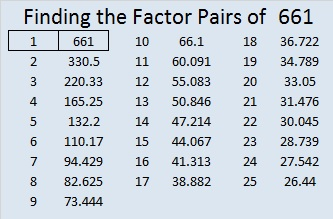# 661 Candy Corn

25² + 6² = 661

661 is the hypotenuse of the primitive Pythagorean triple 300-589-661 which was calculated using 2(25)(6), 25² – 6², 25² + 6².

661 is also the sum of the three prime numbers from 211 to 227. What is the prime number in the middle of the sum?

This Find the Factors puzzle is supposed to look like a piece of candy corn. 🙂Print the puzzles or type the solution on this excel file: 10 Factors 2015-10-26

Here’s the puzzle without the possibly distracting color:————————————

• 661 is a prime number. 659 and 661 are twin primes.
• Prime factorization: 661 is prime.
• The exponent of prime number 661 is 1. Adding 1 to that exponent we get (1 + 1) = 2. Therefore 661 has exactly 2 factors.
• Factors of 661: 1, 661
• Factor pairs: 661 = 1 x 661
• 661 has no square factors that allow its square root to be simplified. √661 ≈ 25.70992.How do we know that 661 is a prime number? If 661 were not a prime number, then it would be divisible by at least one prime number less than or equal to √661 ≈ 25.7. Since 661 cannot be divided evenly by 2, 3, 5, 7, 11, 13, 17, 19, or 23, we know that 661 is a prime number.

Here’s another way we know that 661 is a prime number: Since 25² + 6² = 661, and 25 and 6 have no common prime factors, 661 will be prime unless it is divisible by a primitive Pythagorean hypotenuse less than or equal to √661 ≈ 25.7. Since 661 is not divisible by 5, 13, or 17, we know that 661 is a prime number.

—————————————————————————————————

A Logical Approach to solve a FIND THE FACTORS puzzle: Find the column or row with two clues and find their common factor. (None of the factors are greater than 10.)  Write the corresponding factors in the factor column (1st column) and factor row (top row).  Because this is a level three puzzle, you have now written a factor at the top of the factor column. Continue to work from the top of the factor column to the bottom, finding factors and filling in the factor column and the factor row one cell at a time as you go.